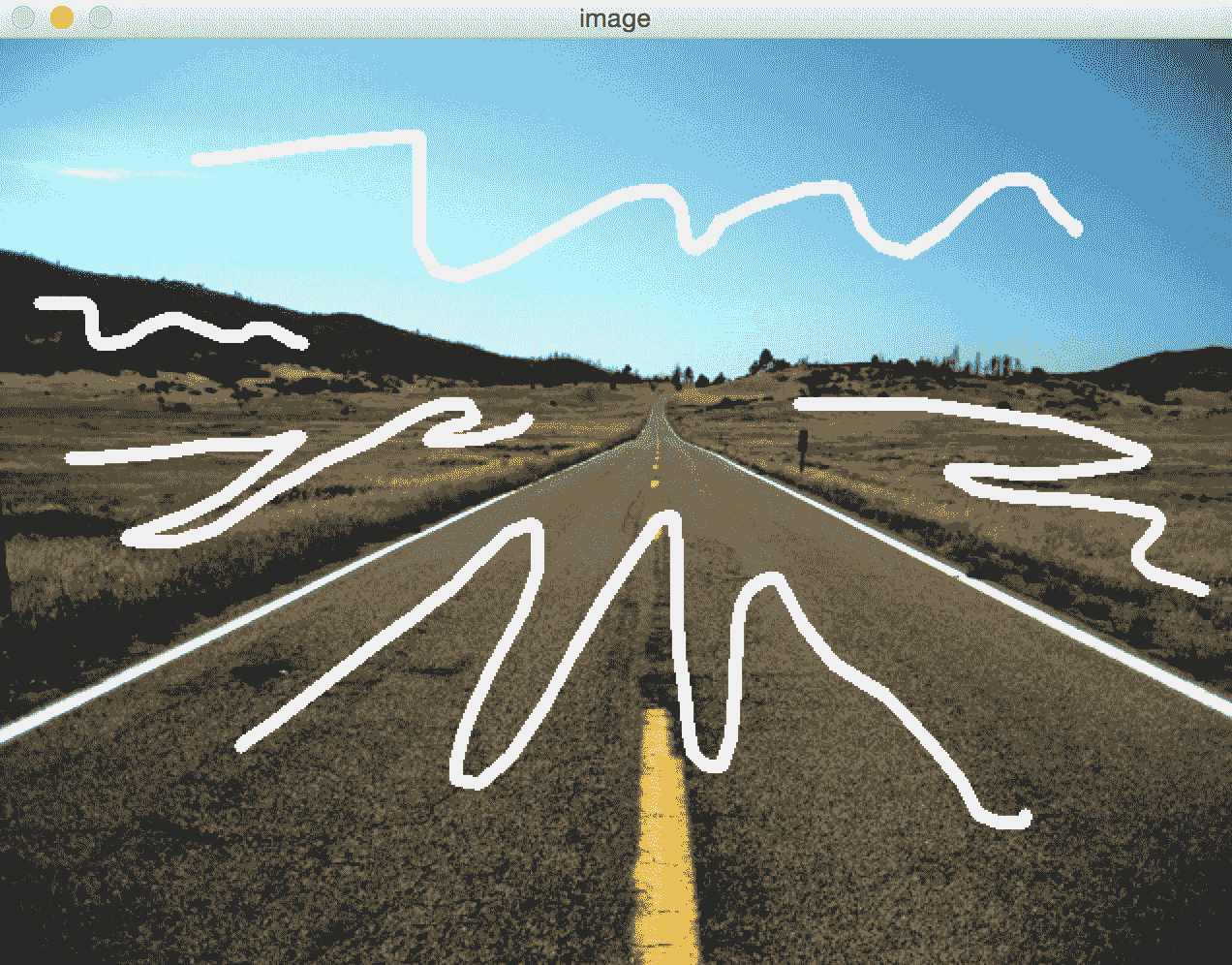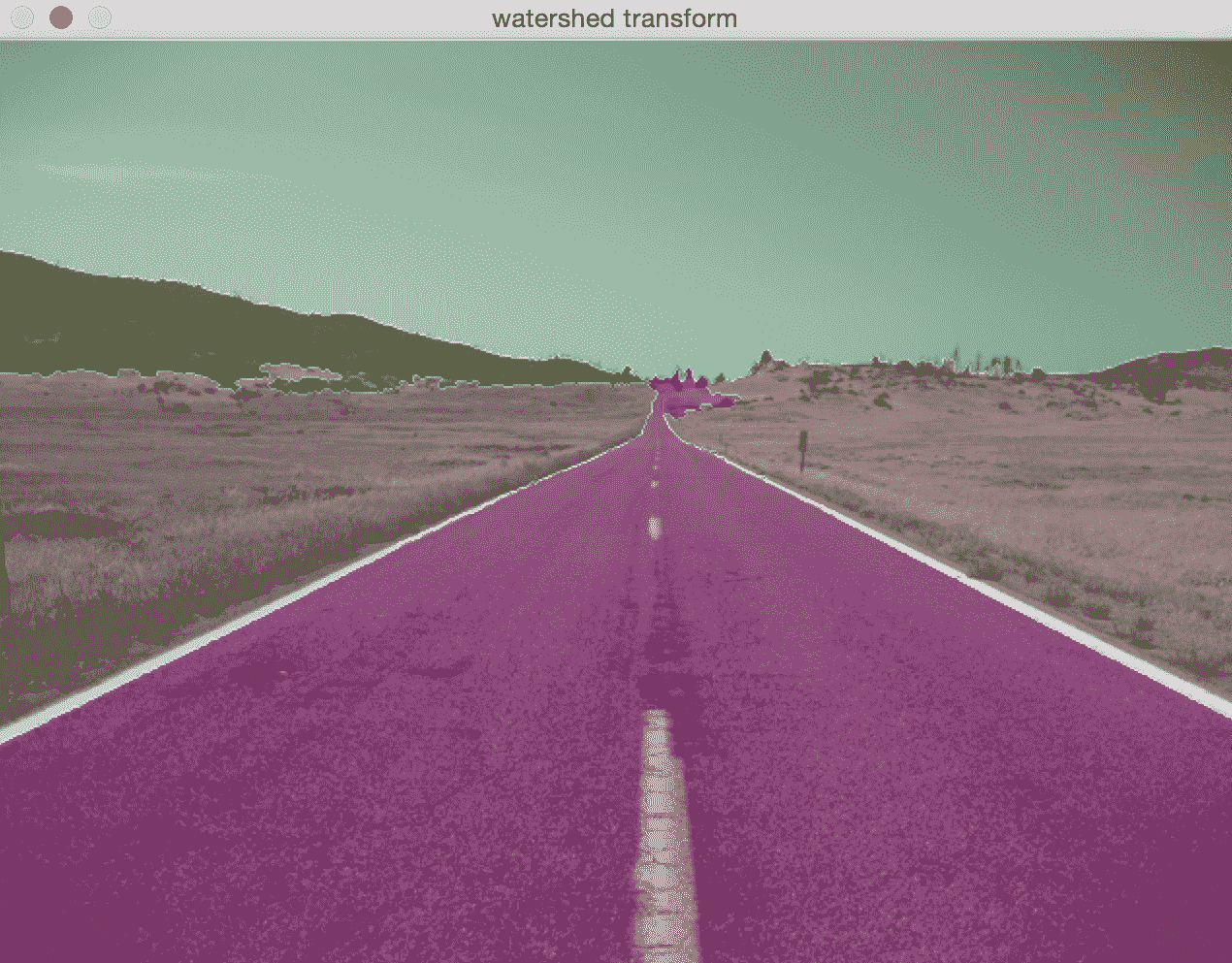# 七、检测形状和分割图像

• 什么是轮廓分析和形状匹配
• 如何匹配形状
• 什么是图像分割
• 如何将图像分割成其组成部分
• 如何将前景与背景分开
• 如何使用各种技术分割图像

# 轮廓分析和形状匹配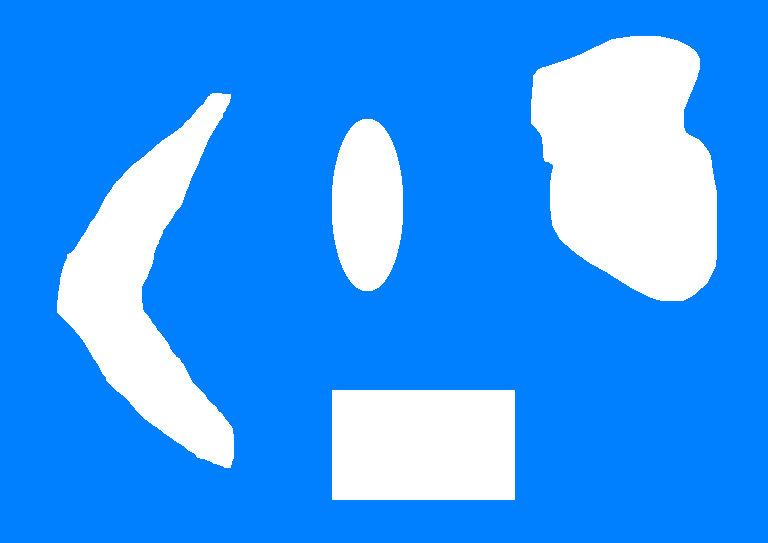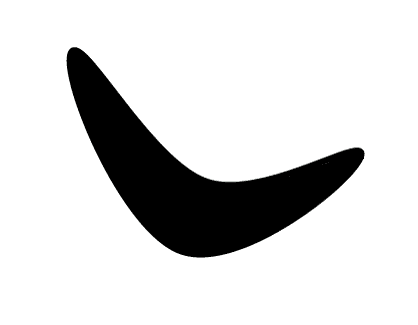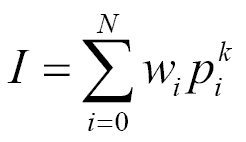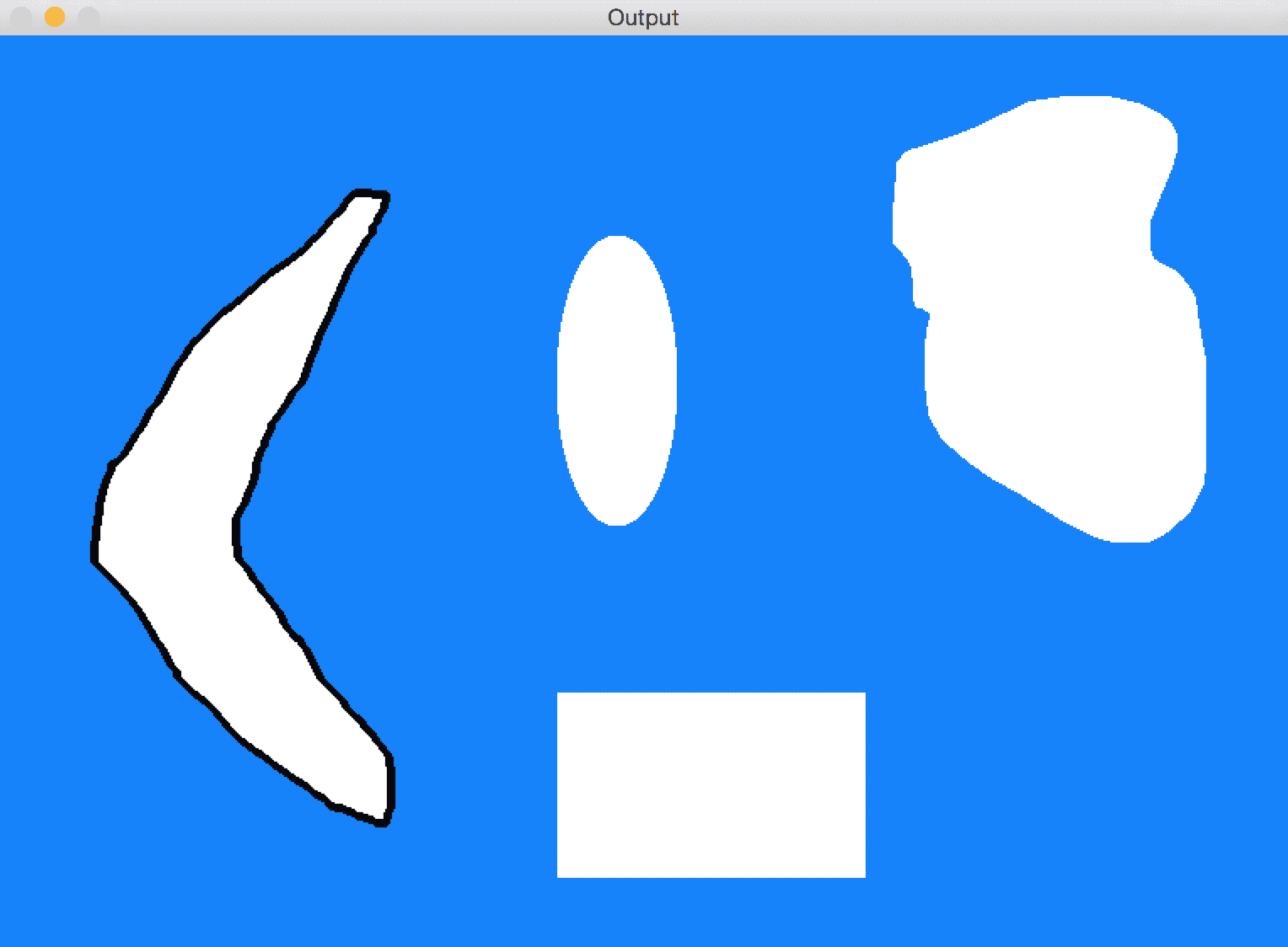import cv2
import numpy as np

# Extract all the contours from the image
def get_all_contours(img):
ref_gray = cv2.cvtColor(img, cv2.COLOR_BGR2GRAY)
ret, thresh = cv2.threshold(ref_gray, 127, 255, 0)
# Find all the contours in the thresholded image. The values
# for the second and third parameters are restricted to a
# certain number of possible values.
im2, contours, hierarchy = cv2.findContours(thresh.copy(), cv2.RETR_LIST, \
cv2.CHAIN_APPROX_SIMPLE )
return contours

# Extract reference contour from the image
def get_ref_contour(img):
contours = get_all_contours(img)

# Extract the relevant contour based on area ratio. We use the
# area ratio because the main image boundary contour is
# extracted as well and we don't want that. This area ratio
# threshold will ensure that we only take the contour inside the image.
for contour in contours:
area = cv2.contourArea(contour)
img_area = img.shape * img.shape
if 0.05 < area/float(img_area) < 0.8:
return contour

if __name__=='__main__':
# Boomerang reference image

# Input image containing all the different shapes

# Extract the reference contour
ref_contour = get_ref_contour(img1)

# Extract all the contours from the input image
input_contours = get_all_contours(img2)

closest_contour = None
min_dist = None
contour_img = img2.copy()
cv2.drawContours(contour_img, input_contours, -1, color=(0,0,0), thickness=3)
cv2.imshow('Contours', contour_img)
# Finding the closest contour
for contour in input_contours:
# Matching the shapes and taking the closest one using
# Comparison method CV_CONTOURS_MATCH_I3 (second argument)
ret = cv2.matchShapes(ref_contour, contour, 3, 0.0)
print("Contour %d matchs in %f" % (i, ret))
if min_dist is None or ret < min_dist:
min_dist = ret
closest_contour = contour

cv2.drawContours(img2, [closest_contour], 0 , color=(0,0,0), thickness=3)
cv2.imshow('Best Matching', img2)
cv2.waitKey()


matchShapes方法的使用可能与胡矩不变量（CV_CONTOUR_MATCH_I1,2,3）不同，后者由于轮廓的大小，方向或旋转而可能产生不同的最佳匹配形状。 要了解更多信息，可以在这个页面上查看官方文档。

# 近似轮廓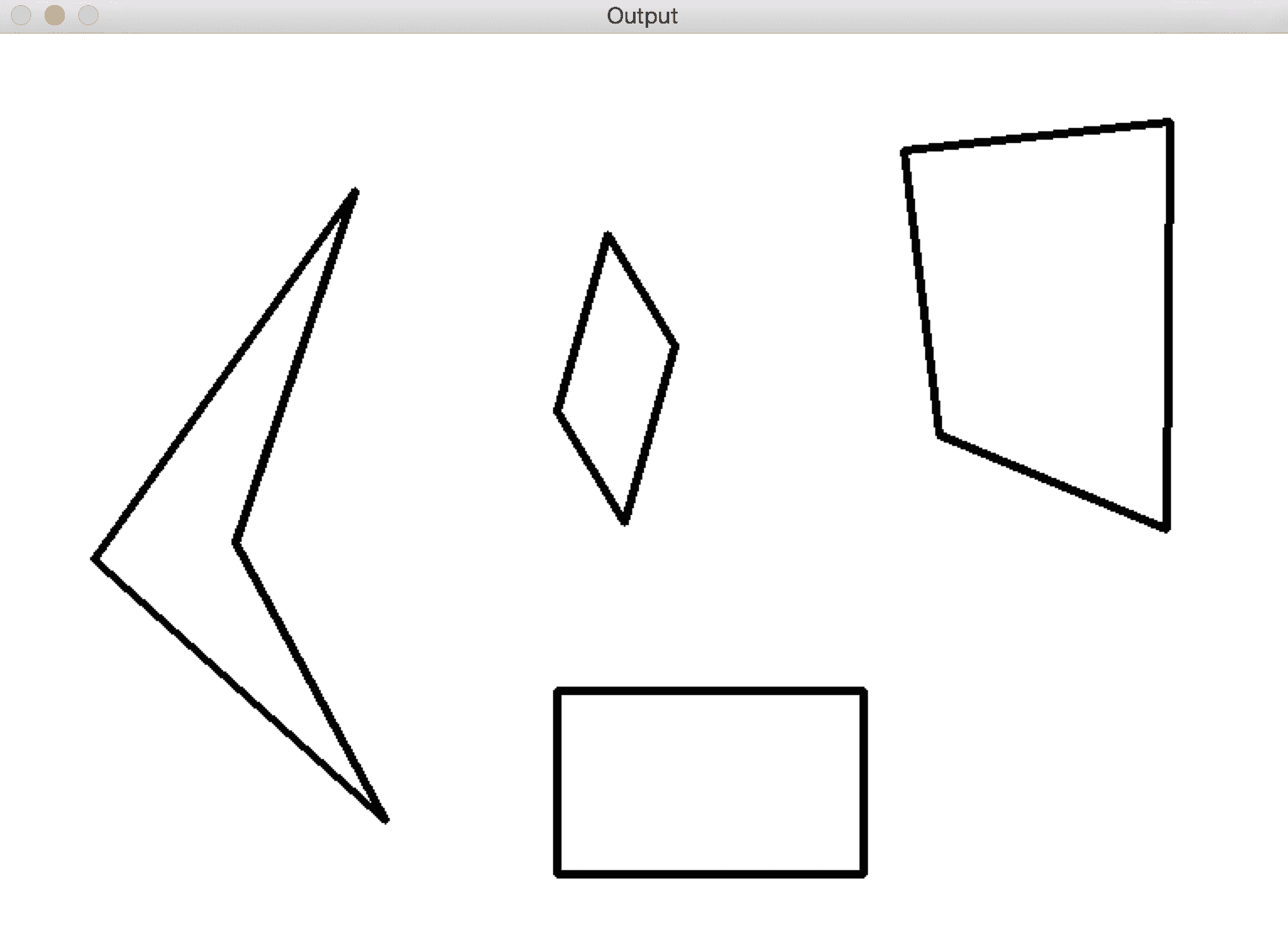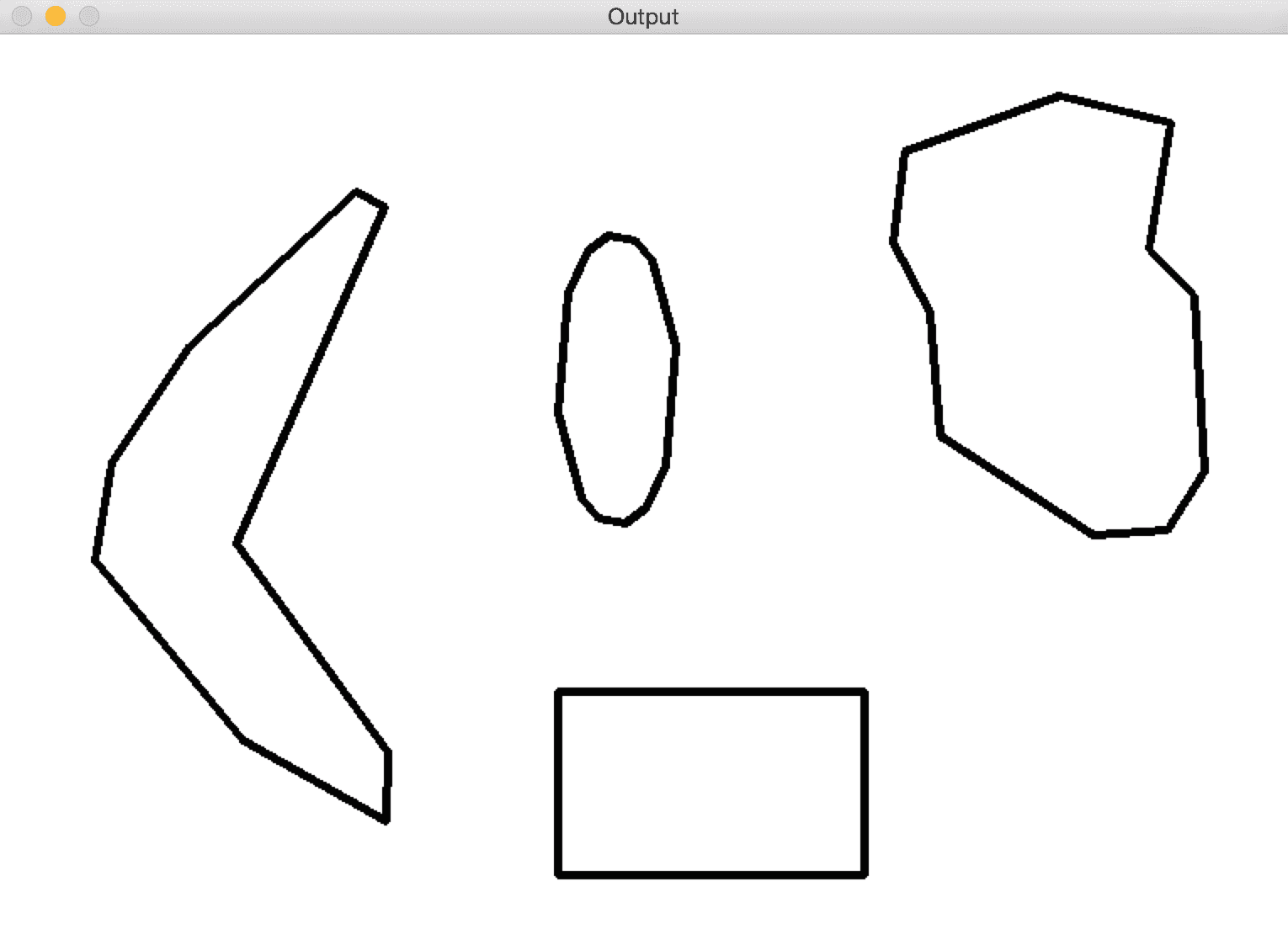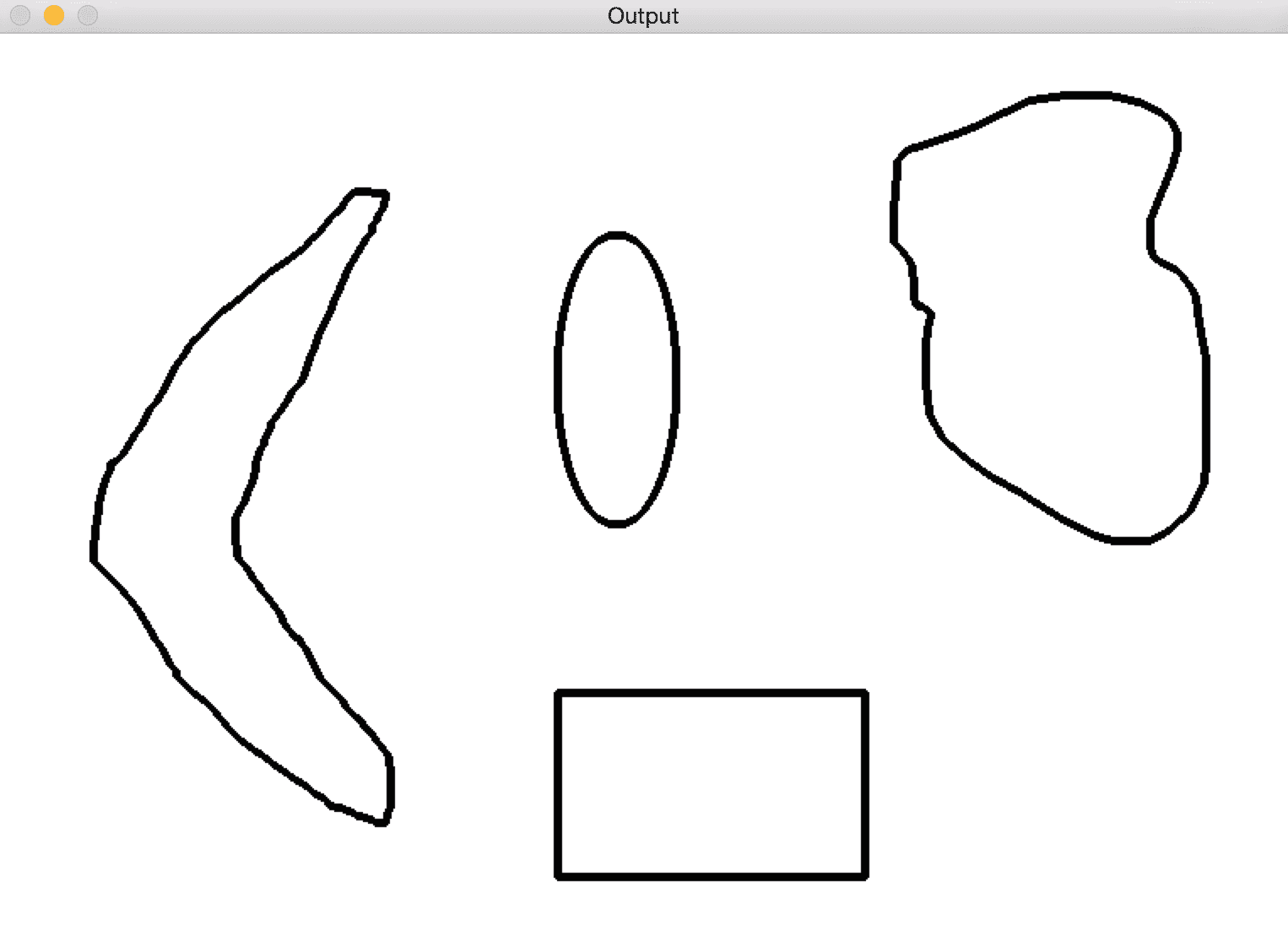import sys
import cv2
import numpy as np

if __name__=='__main__':
# Input image containing all the different shapes
# Extract all the contours from the input image
input_contours = get_all_contours(img1)

contour_img = img1.copy()
smoothen_contours = []
factor = 0.05

# Finding the closest contour
for contour in input_contours:
epsilon = factor * cv2.arcLength(contour, True)
smoothen_contours.append(cv2.approxPolyDP(contour, epsilon, True))

cv2.drawContours(contour_img, smoothen_contours, -1, color=(0,0,0), thickness=3)
cv2.imshow('Contours', contour_img)
cv2.waitKey()


# 识别出一片突出的披萨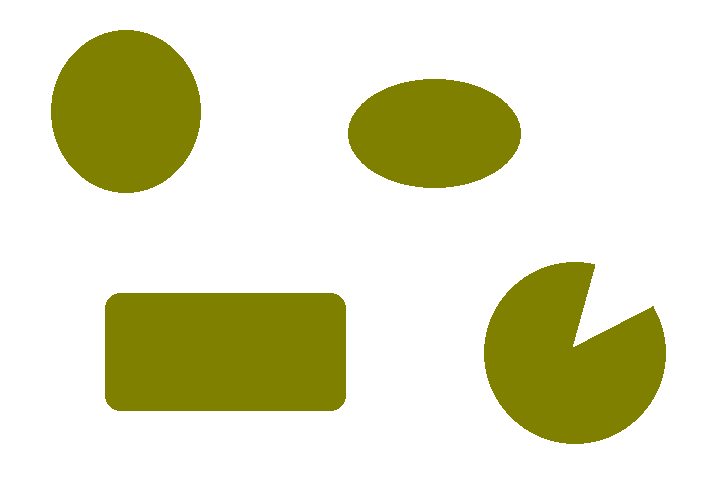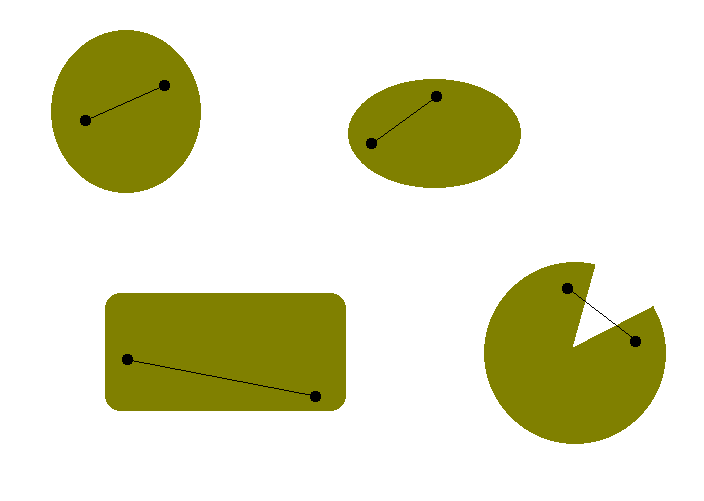import sys
import cv2
import numpy as np

if __name__=='__main__':

# Iterate over the extracted contours
# Using previous get_all_contours() method
for contour in get_all_contours(img):
# Extract convex hull from the contour
hull = cv2.convexHull(contour, returnPoints=False)

# Extract convexity defects from the above hull
# Being a convexity defect the cavities in the hull segments
defects = cv2.convexityDefects(contour, hull)

if defects is None:
continue

# Draw lines and circles to show the defects
for i in range(defects.shape):
start_defect, end_defect, far_defect, _ = defects[i,0]
start = tuple(contour[start_defect])
end = tuple(contour[end_defect])
far = tuple(contour[far_defect])
cv2.circle(img, far, 5, [128,0,0], -1)
cv2.drawContours(img, [contour], -1, (0,0,0), 3)

cv2.imshow('Convexity defects',img)
cv2.waitKey(0)
cv2.destroyAllWindows()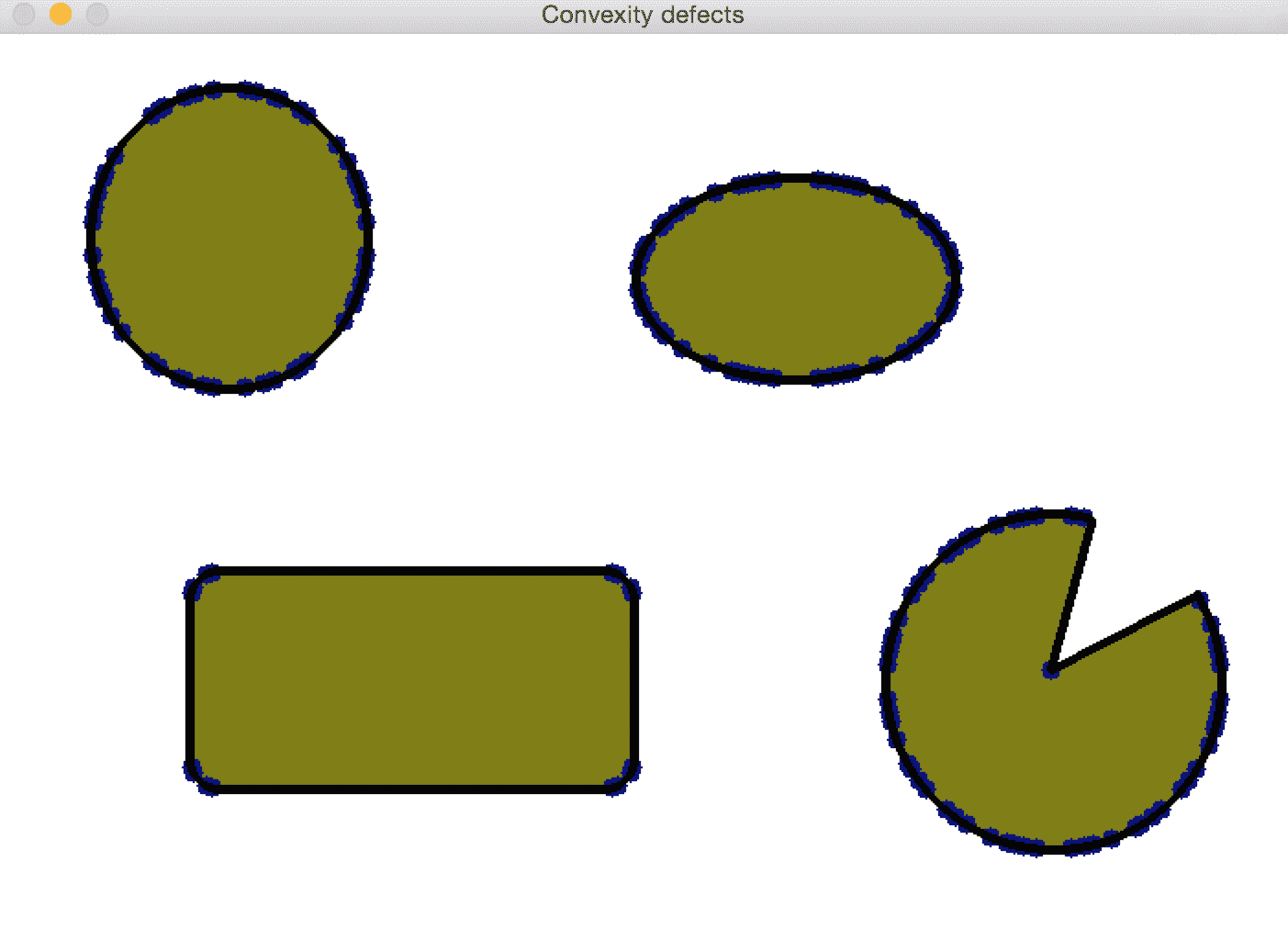factor = 0.01
epsilon = factor * cv2.arcLength(contour, True)
contour = cv2.approxPolyDP(contour, epsilon, True)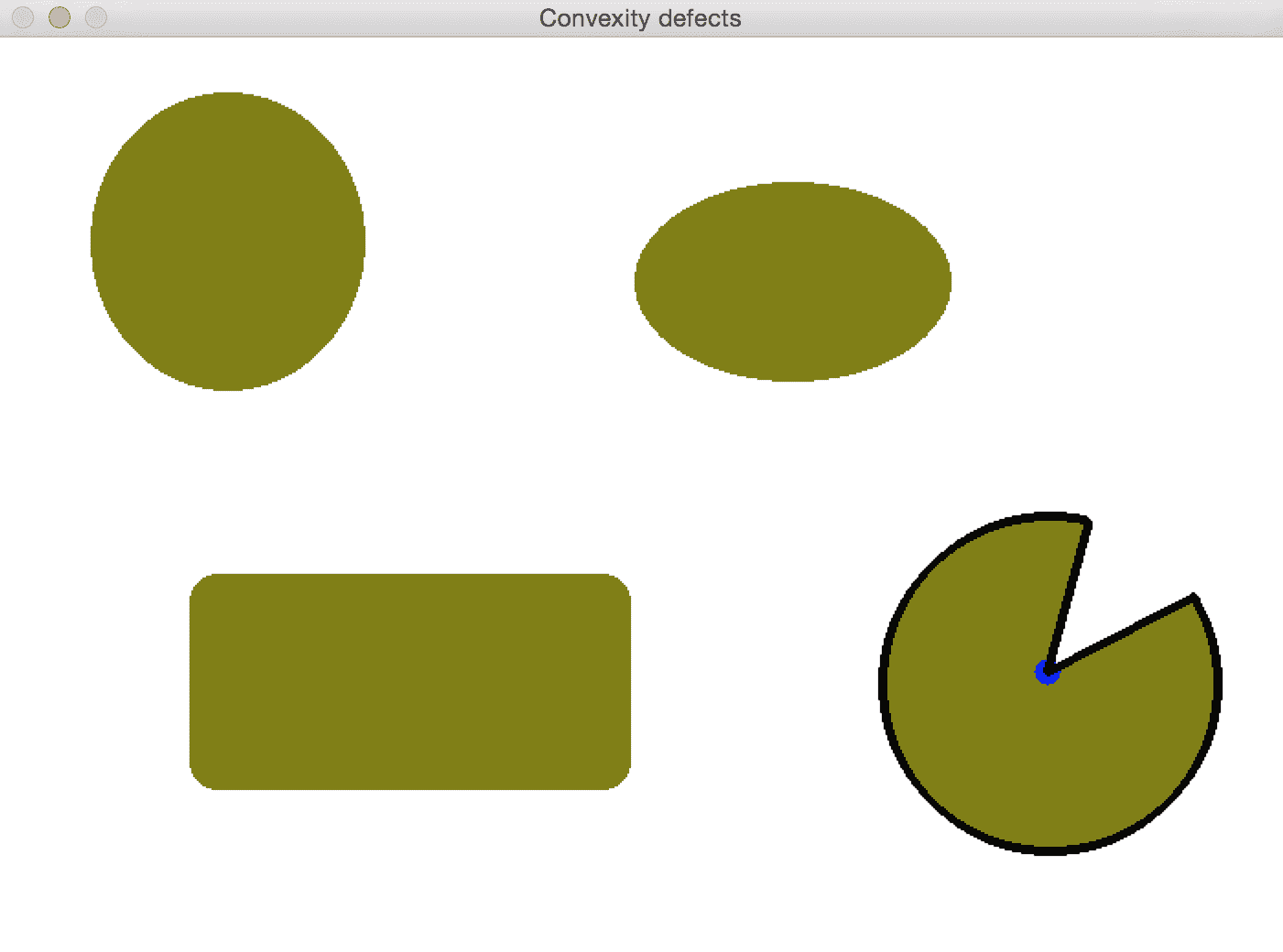# 如何检查形状？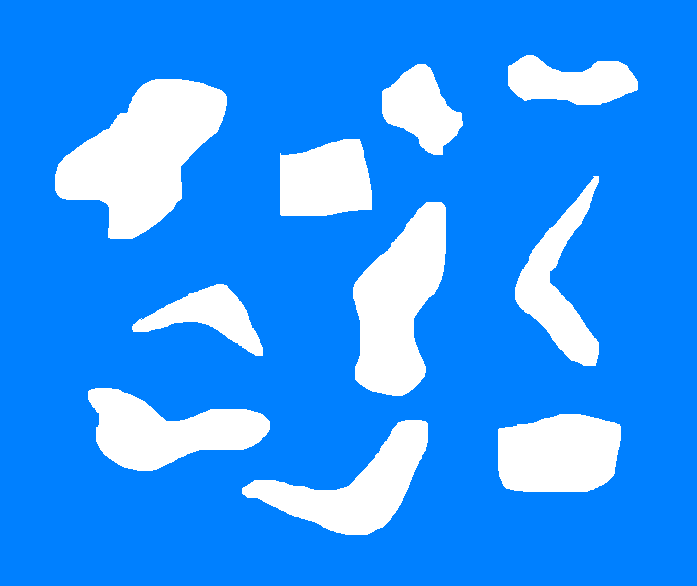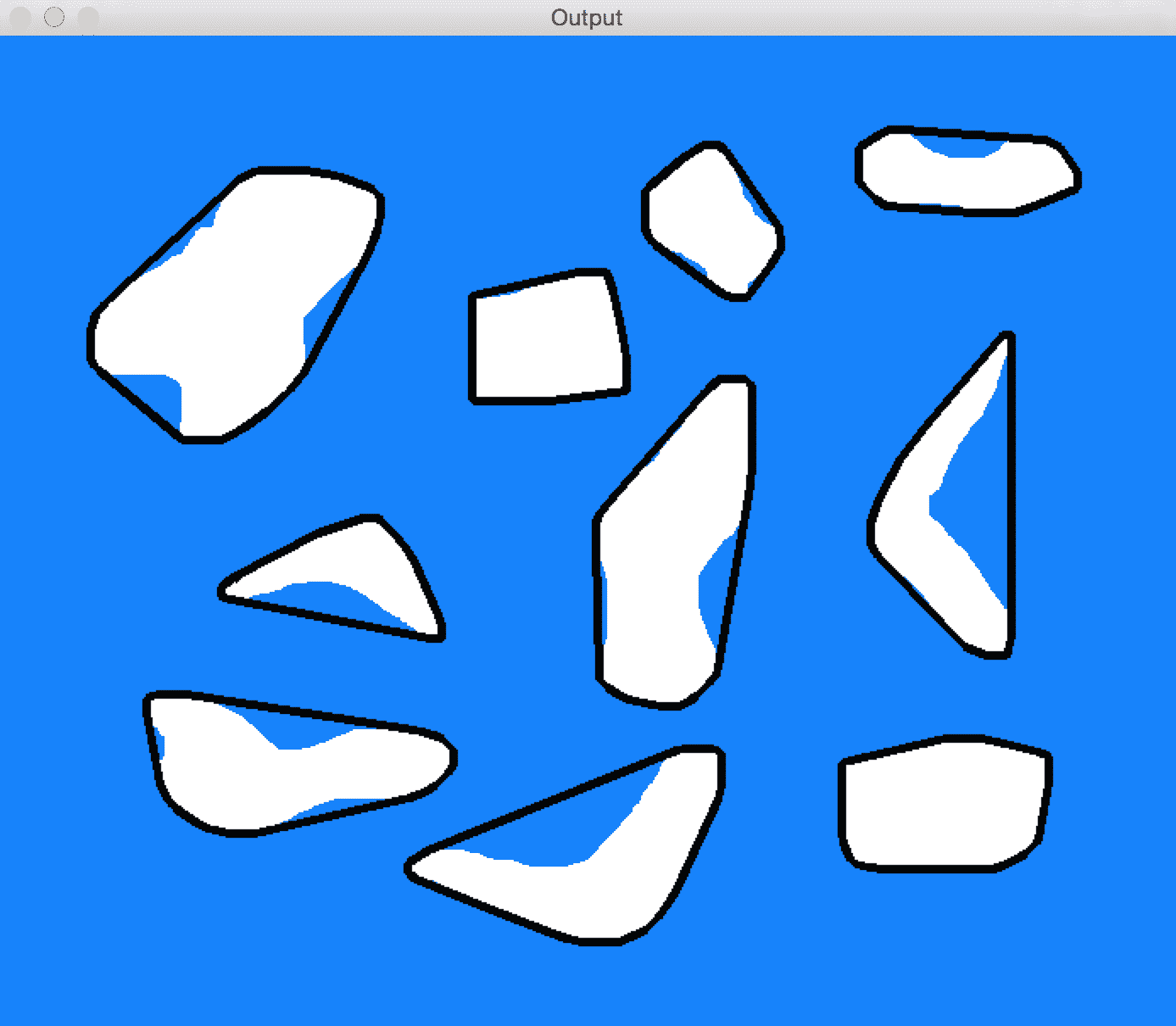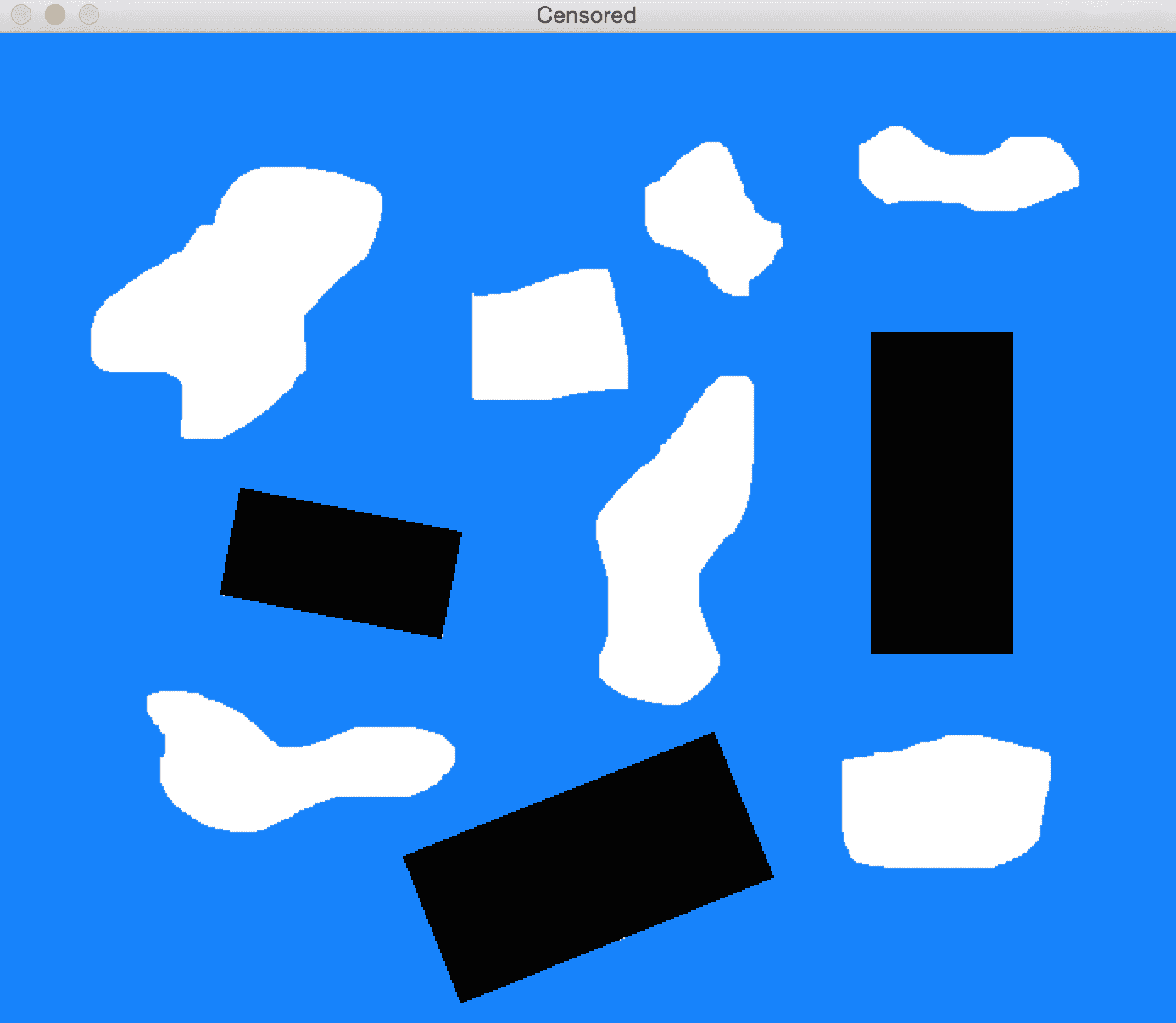import sys
import cv2
import numpy as np

if __name__=='__main__':
# Input image containing all the shapes

img_orig = np.copy(img)
input_contours = get_all_contours(img)
solidity_values = []

# Compute solidity factors of all the contours
for contour in input_contours:
area_contour = cv2.contourArea(contour)
convex_hull = cv2.convexHull(contour)
area_hull = cv2.contourArea(convex_hull)
solidity = float(area_contour)/area_hull
solidity_values.append(solidity)

# Clustering using KMeans
criteria = (cv2.TERM_CRITERIA_EPS + cv2.TERM_CRITERIA_MAX_ITER, 10, 1.0)
flags = cv2.KMEANS_RANDOM_CENTERS
solidity_values = \               np.array(solidity_values).reshape((len(solidity_values),1)).astype('float32')
compactness, labels, centers = cv2.kmeans(solidity_values, 2, None, criteria, 10, flags)

closest_class = np.argmin(centers)
output_contours = []
for i in solidity_values[labels==closest_class]:
index = np.where(solidity_values==i)
output_contours.append(input_contours[index])

cv2.drawContours(img, output_contours, -1, (0,0,0), 3)
cv2.imshow('Output', img)

# Censoring
for contour in output_contours:
rect = cv2.minAreaRect(contour)
box = cv2.boxPoints(rect)
box = np.int0(box)
cv2.drawContours(img_orig, [box], 0, (0,0,0), -1)

cv2.imshow('Censored', img_orig)
cv2.waitKey()


# 什么是图像分割？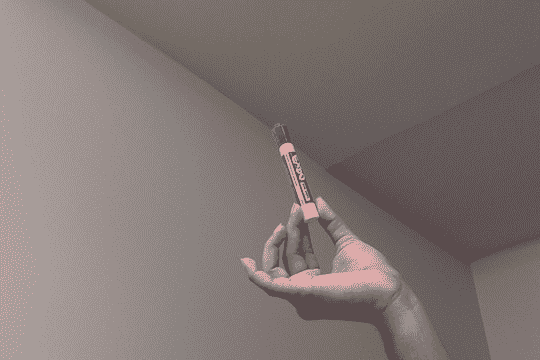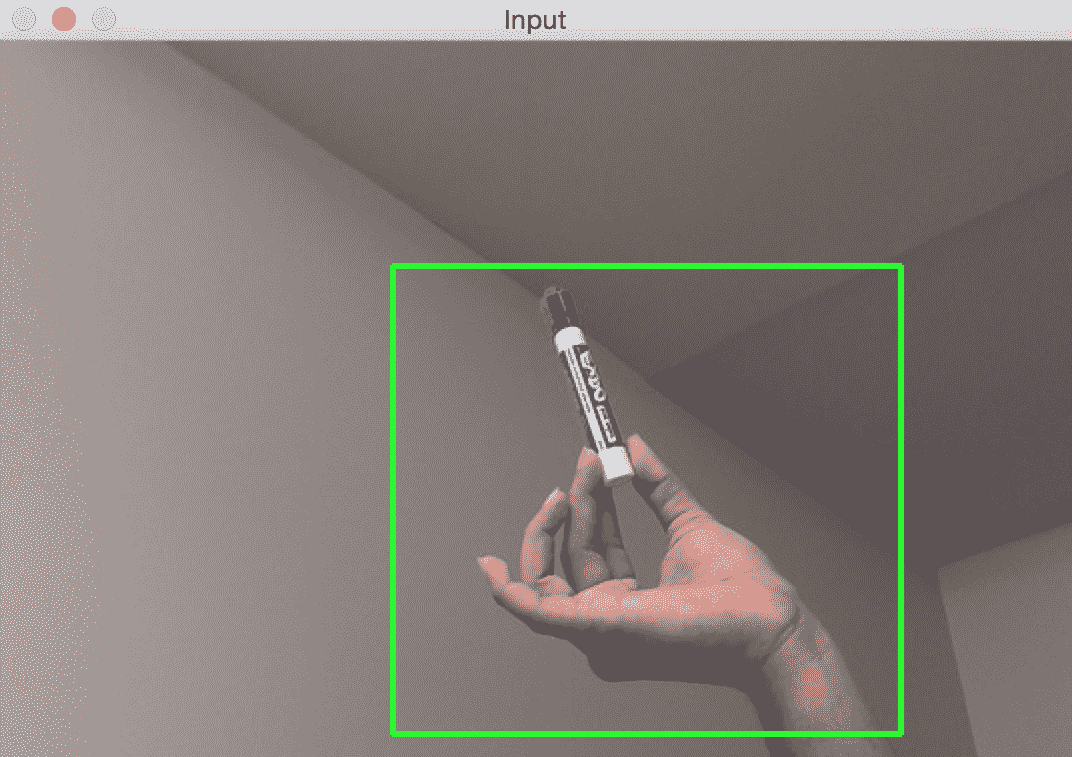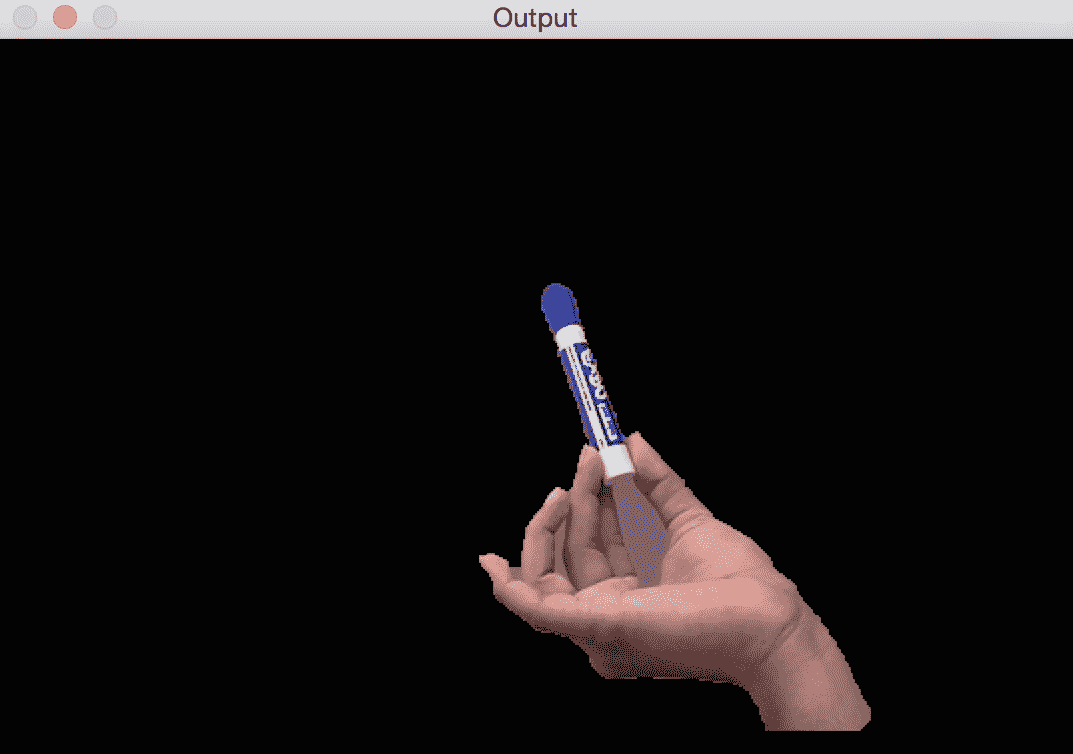import sys
import cv2
import numpy as np

# Draw rectangle based on the input selection
def draw_rectangle(event, x, y, flags, params):
global x_init, y_init, drawing, top_left_pt, bottom_right_pt, img_orig

# Detecting mouse button down event
if event == cv2.EVENT_LBUTTONDOWN:
drawing = True
x_init, y_init = x, y

# Detecting mouse movement
elif event == cv2.EVENT_MOUSEMOVE:
if drawing:
top_left_pt, bottom_right_pt = (x_init,y_init), (x,y)
img[y_init:y, x_init:x] = 255 - img_orig[y_init:y, x_init:x]
cv2.rectangle(img, top_left_pt, bottom_right_pt, (0,255,0), 2)

# Detecting mouse button up event
elif event == cv2.EVENT_LBUTTONUP:
drawing = False
top_left_pt, bottom_right_pt = (x_init,y_init), (x,y)
img[y_init:y, x_init:x] = 255 - img[y_init:y, x_init:x]
cv2.rectangle(img, top_left_pt, bottom_right_pt, (0,255,0), 2)
rect_final = (x_init, y_init, x-x_init, y-y_init)

# Run Grabcut on the region of interest
run_grabcut(img_orig, rect_final)

# Grabcut algorithm
def run_grabcut(img_orig, rect_final):

# Extract the rectangle and set the region of
# interest in the above mask
x,y,w,h = rect_final

# Initialize background and foreground models
bgdModel = np.zeros((1,65), np.float64)
fgdModel = np.zeros((1,65), np.float64)

# Run Grabcut algorithm
cv2.grabCut(img_orig, mask, rect_final, bgdModel, fgdModel, 5, cv2.GC_INIT_WITH_RECT)

# Apply the above mask to the image

# Display the image
cv2.imshow('Output', img_orig)

if __name__=='__main__':
drawing = False
top_left_pt, bottom_right_pt = (-1,-1), (-1,-1)

img = img_orig.copy()

cv2.namedWindow('Input')
cv2.setMouseCallback('Input', draw_rectangle)

while True:
cv2.imshow('Input', img)
c = cv2.waitKey(1)
if c == 27:
break

cv2.destroyAllWindows()


# 分水岭算法

OpenCV 随附分水岭算法的默认实现，该理论认为，任何灰度图像都可以被视为地形表面，高强度表示山峰和丘陵，而低强度表示山谷。 该算法非常有名，并且有很多实现方式。